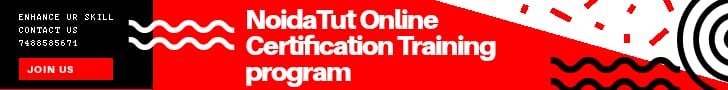FREE E LEARNING PLATFORM

# Check whether an input number is Prime or notA number which is only divisible by itself and 1 is known as prime number, for example: 5 is a prime number because it is only divisible by itself and 1.

This program takes the value of num (entered by user) and checks whether the num is prime number or not.

### Example: Check whether number is prime number or not

```#include <iostream>
using namespace std;
int main(){
int num;
bool flag = true;
cout<<"Enter any number(should be positive integer): ";
cin>>num;       for(int i = 2; i <= num / 2; i++) {
if(num % i == 0) {
flag = false;
break;
}     }
if (flag==true)
cout<<num<<" is a prime number";
else
cout<<num<<" is not a prime number";
return 0;
}```

### Output

```Enter any number(should be positive integer): 149
149 is a prime number```

You can also use while loop to solve this problem, just replace the following code in above program:

```for(int i = 2; i <= num / 2; i++) {
if(num % i == 0) {
flag = false;
break;
}  }

```

with this code:

```int i=2;
while(i<=num/2){
if(num % i == 0)
{
flag = false;
break;
}
i++;
}```

### Example: Check whether number is prime number or not using while loop

```#include <iostream>
using namespace std;
int main(){
int num;
bool flag = true;
cout<<"Enter any number(should be positive integer): ";
cin>>num;
int i=2;
while(i<=num/2){
if(num % i == 0)
{
flag = false;
break;
}
i++;
}
if (flag==true)
cout<<num<<" is a prime number";
else
cout<<num<<" is not a prime number";
return 0;
}```